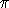## CGAL::Circle_3<Kernel>

### Definition

An object of type Circle_3<Kernel> is a circle in the three-dimensional Euclidean space3. Note that the circle can be degenerate, i.e. the squared radius may be zero.

### Creation

Circle_3<Kernel> c ( Point_3<Kernel> center, Kernel::FT sq_r, Plane_3<Kernel> plane);
introduces a variable c of type Circle_3<Kernel>. It is initialized to the circle of center center and squared radius sq_r in plane plane.
 Precondition: center lies in plane and sq_r0.

Circle_3<Kernel> c ( Point_3<Kernel> center, Kernel::FT sq_r, Vector_3<Kernel> n);
introduces a variable c of type Circle_3<Kernel>. It is initialized to the circle of center center and squared radius sq_r in a plane normal to the vector n.
 Precondition: sq_r0.

Circle_3<Kernel> c ( Point_3<Kernel> p, Point_3<Kernel> q, Point_3<Kernel> r);
introduces a variable c of type Circle_3<Kernel>. It is initialized to the circle passing through the three points.
 Precondition: The three points are not collinear.

Circle_3<Kernel> c ( Sphere_3<Kernel> sphere1, Sphere_3<Kernel> sphere2);
introduces a variable c of type Circle_3<Kernel>. It is initialized to the circle along which the two spheres intersect.
 Precondition: The two spheres intersect along a circle.

Circle_3<Kernel> c ( Sphere_3<Kernel> sphere, Plane_3<Kernel> plane);
introduces a variable c of type Circle_3<Kernel>. It is initialized to the circle along which the sphere and the plane intersect.
 Precondition: The sphere and the plane intersect along a circle.

Circle_3<Kernel> c ( Plane_3<Kernel> plane, Sphere_3<Kernel> sphere);
introduces a variable c of type Circle_3<Kernel>. It is initialized to the circle along which the sphere and the plane intersect.
 Precondition: The sphere and the plane intersect along a circle.

### Access Functions

 Point_3 c.center () returns the center of c. Kernel::FT c.squared_radius () returns the squared radius of c. Plane_3 c.supporting_plane () returns the supporting plane of c. Sphere_3 c.diametral_sphere () returns the diametral sphere of c. Kernel::FT c.area_divided_by_pi () returns the area of c, divided by. double c.approximate_area () returns an approximation of the area of c. Kernel::FT c.squared_length_divided_by_pi_square () returns the squared length of c, divided by2. double c.approximate_squared_length () returns an approximation of the squared length (i.e. perimeter) of c.

### Predicates

 bool c.has_on ( Point_3 p)

### Operations

 bool c.operator == ( c1, c2) returns true, iff c1 and c2 are equal, i.e. if they have the same center, the same squared radius and the same supporting plane. bool c.operator != ( c1, c2) Bbox_3 c.bbox () returns a bounding box containing c.